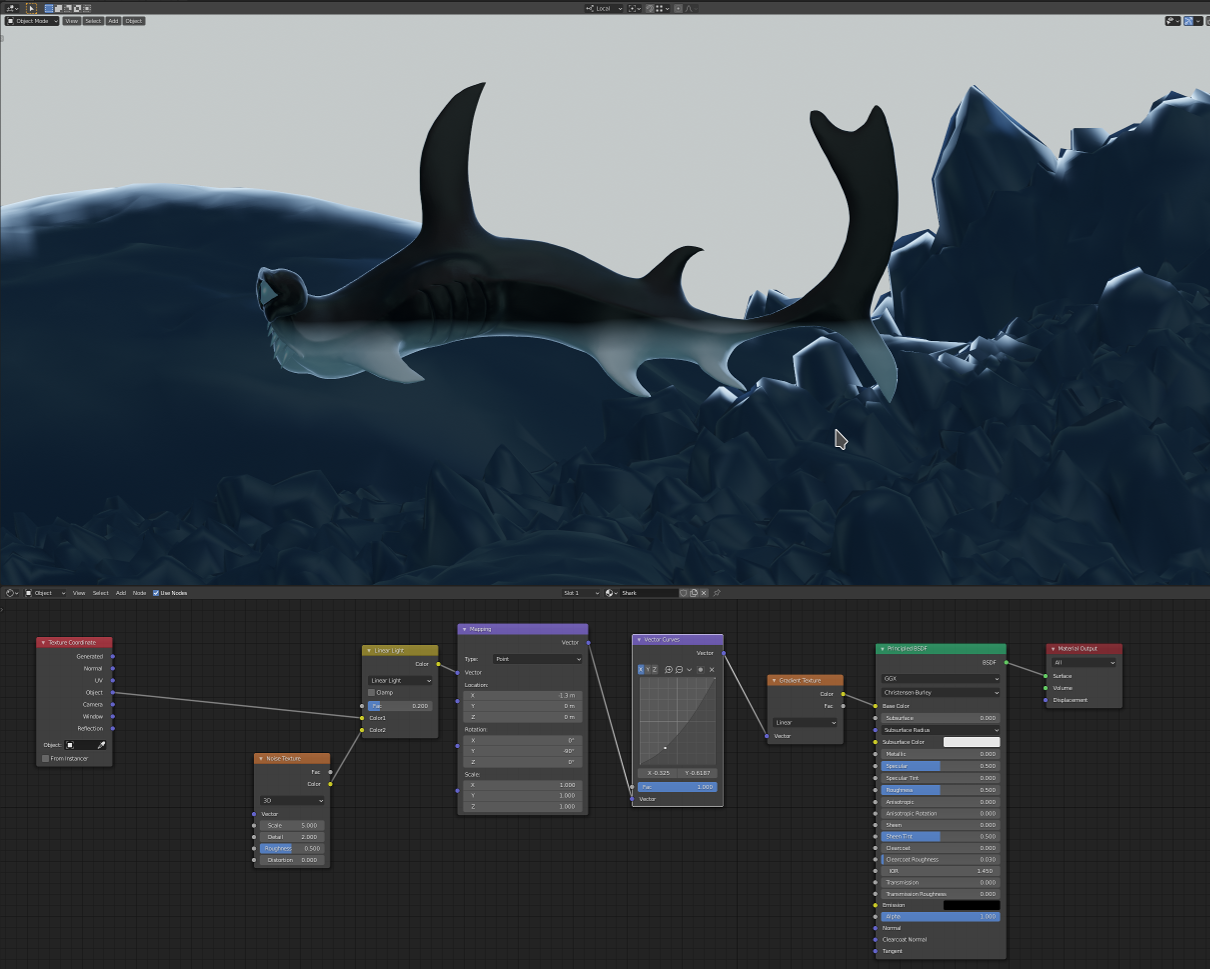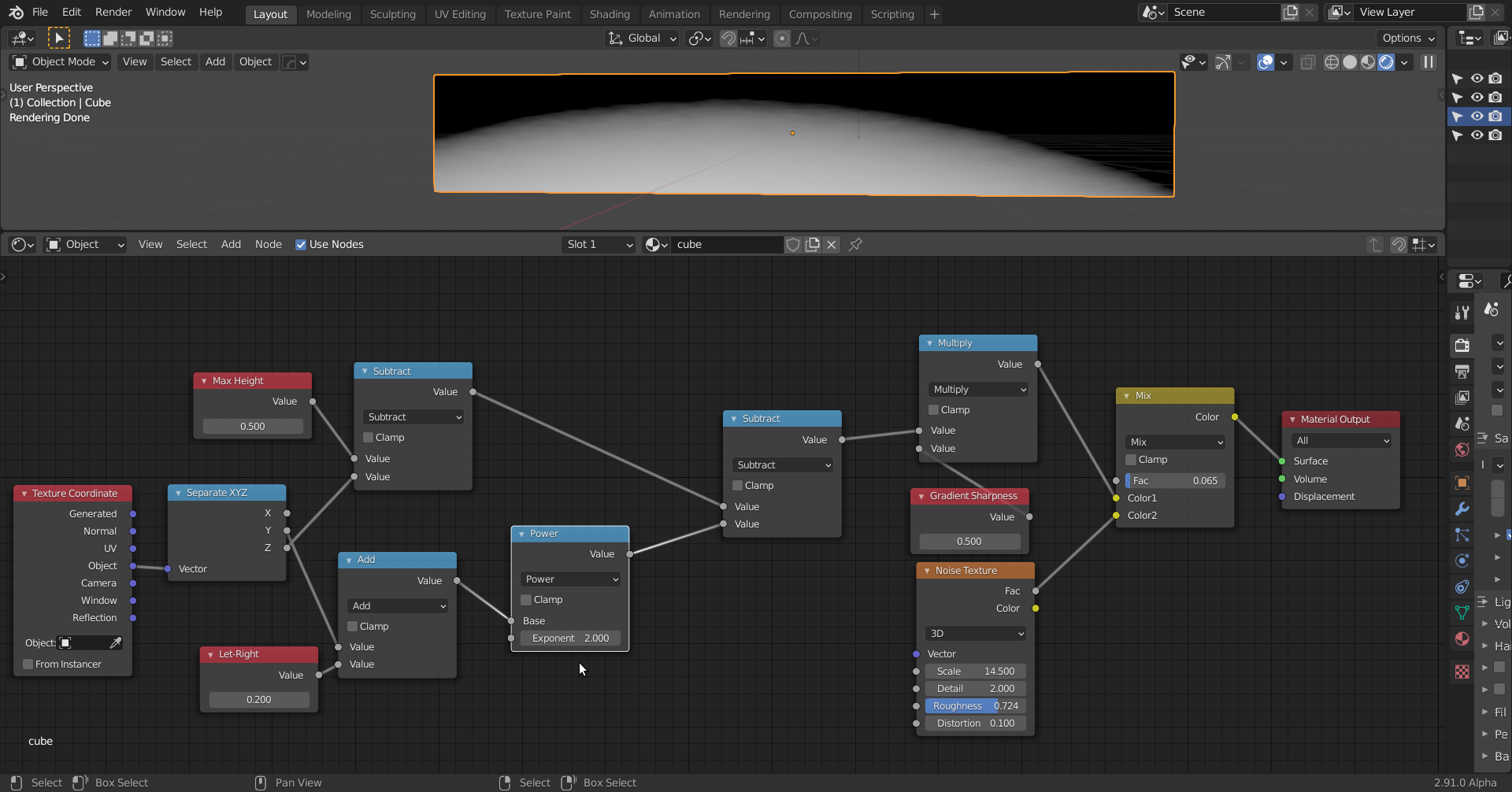## Is there a way to make the gradient curved instead of straight

I did the hammerhead shark and the way it's laid out the body is pretty curved.

I was wondering if there was a way to make the texture gradient follow a curve instead of a straight line.• Sure:Here the shape of the curvature is made with a basic quadratiic equation:Z is dependent on x² (Power Node).

By changing the equation to a higher degree polynomial, you can get more 'waves' (ie: more 'max and mins')

Try to understand what is happening  and then ask questions if something is not clear ;)

• Spikey, you math so much harder than me. Delivering elegant solutions that are beyond me. Never ceases to impress me, sir 👏

• Or,using a cubic:Just fill in the Y values where the Z should be zero (or a, if you change the Height to a).

I left out the Noise here, for clarity ;)

• Thanks Spikeyxxx!  I appreciate it!

I'll give one or both of these a try.